#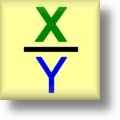Fractions Worksheets

## Printable Fractions Worksheets for Teachers

Here is a graphic preview for all of the fractions worksheets. You can select different variables to customize these fractions worksheets for your needs. The fractions worksheets are randomly created and will never repeat so you have an endless supply of quality fractions worksheets to use in the classroom or at home. Our fractions worksheets are free to download, easy to use, and very flexible.

If you're looking for a great tool for adding, subtracting, multiplying or dividing mixed fractions check out this online Fraction Calculator.

## Quick Link for All Fractions Worksheets

Click the image to be taken to that Fractions Worksheet.

##### Visual Aids for TeachingFractions Worksheets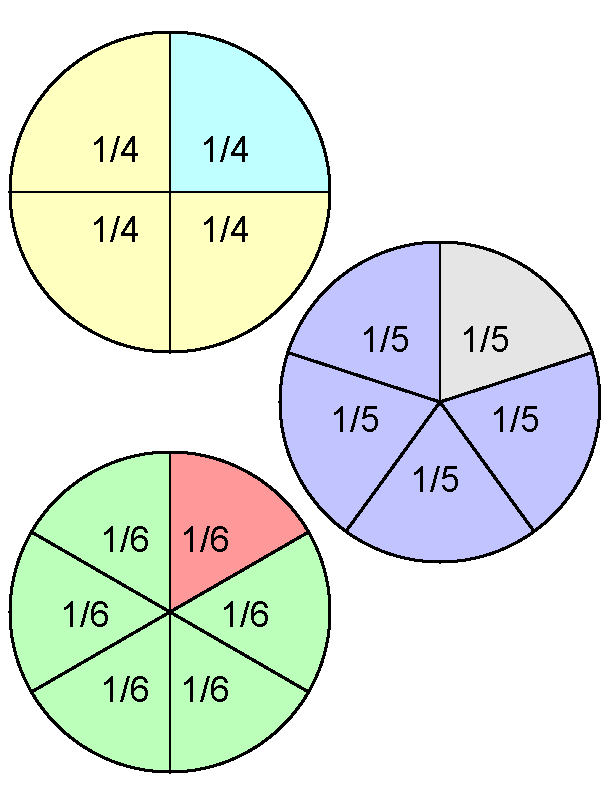##### Visual Fraction WorksheetsFractions Worksheets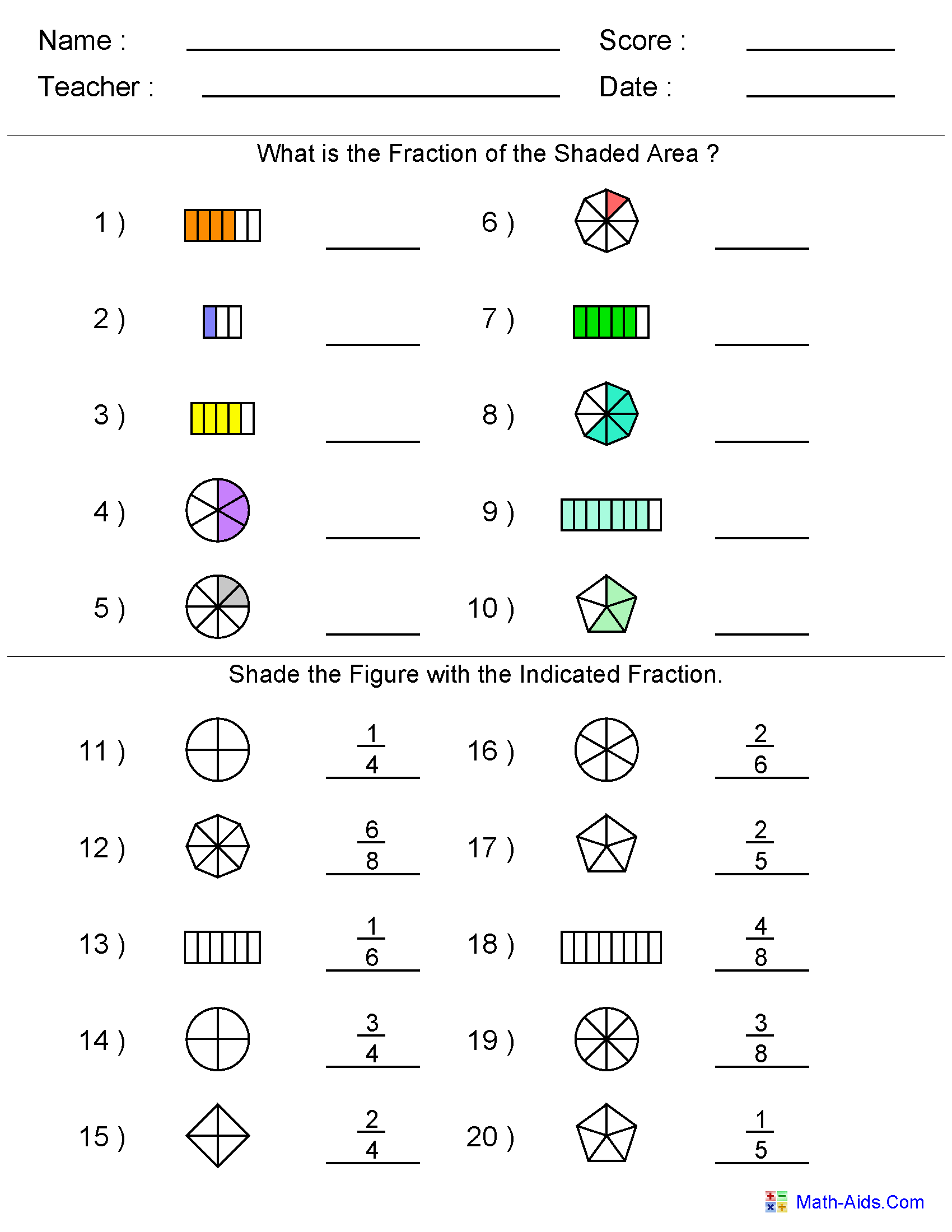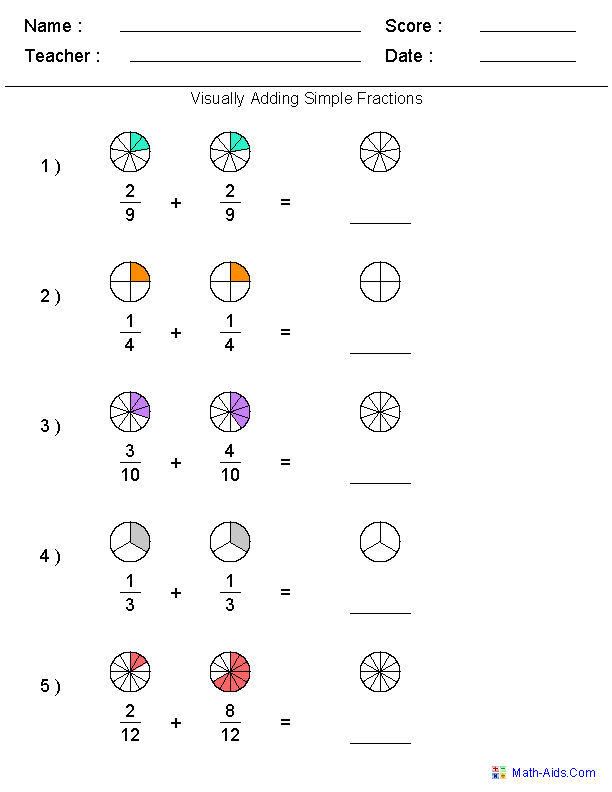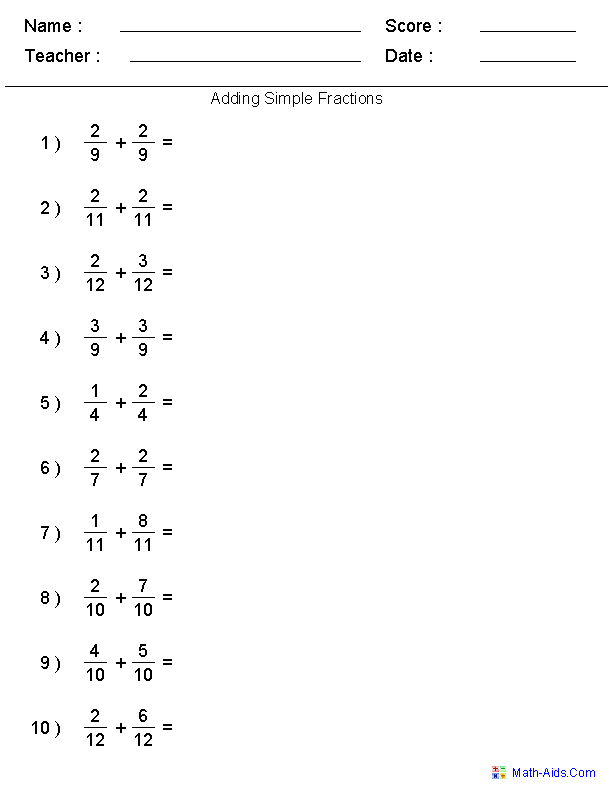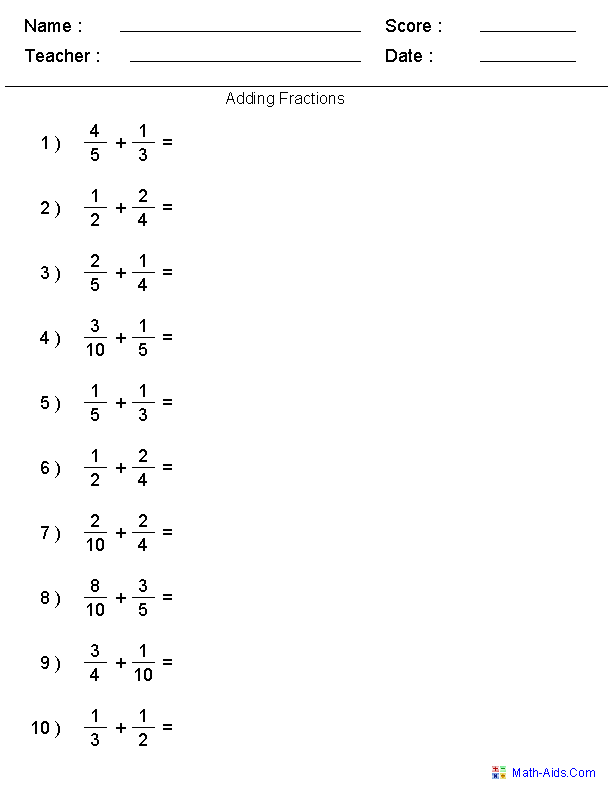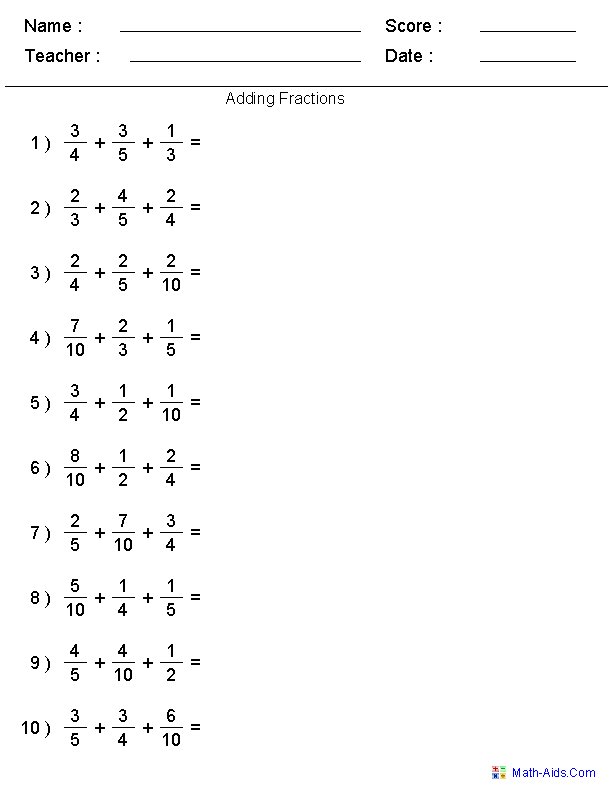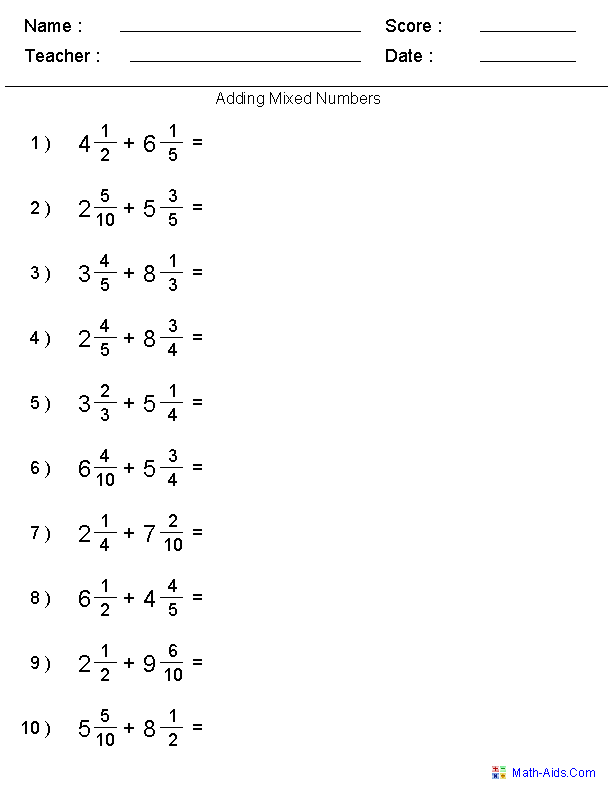##### Visual SubtractionFractions Worksheets##### Subtracting Simple FractionsFractions Worksheets##### Subtracting FractionsFractions Worksheets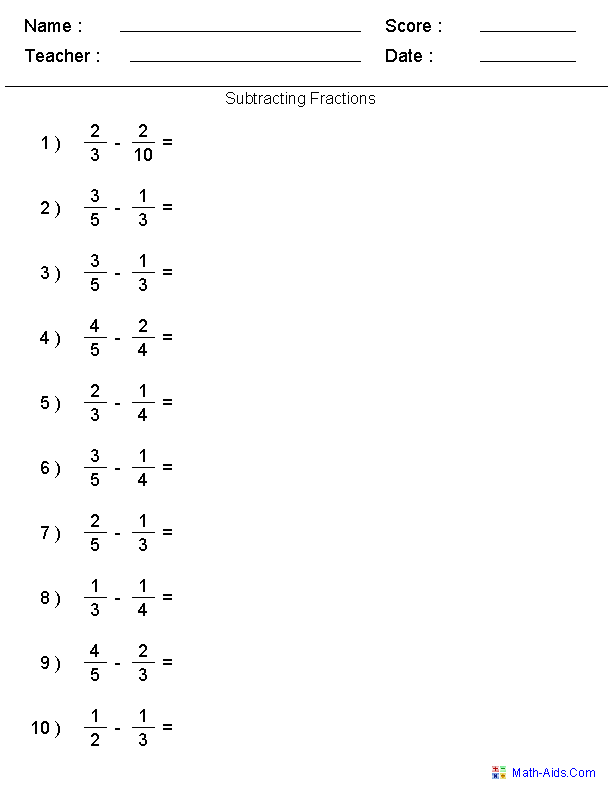##### Subtracting Three FractionsFractions Worksheets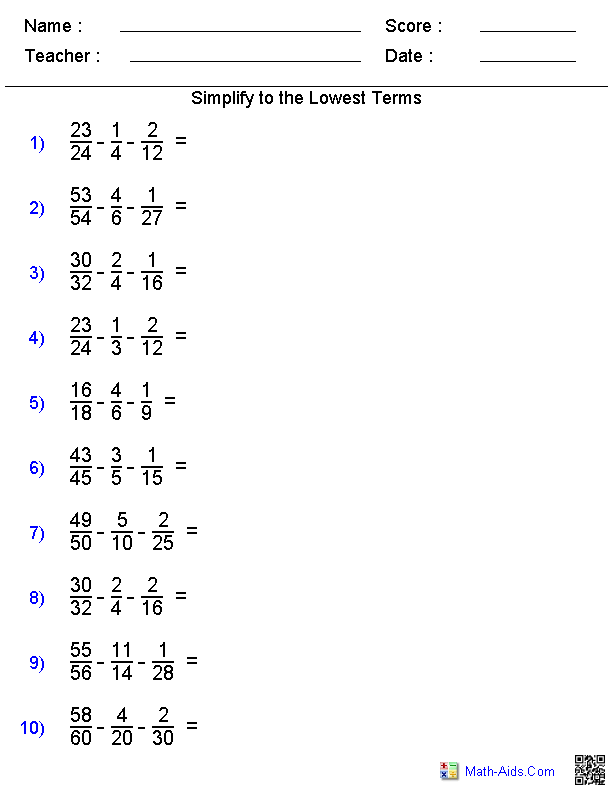##### Subtracting Fractions andWhole Numbers Worksheets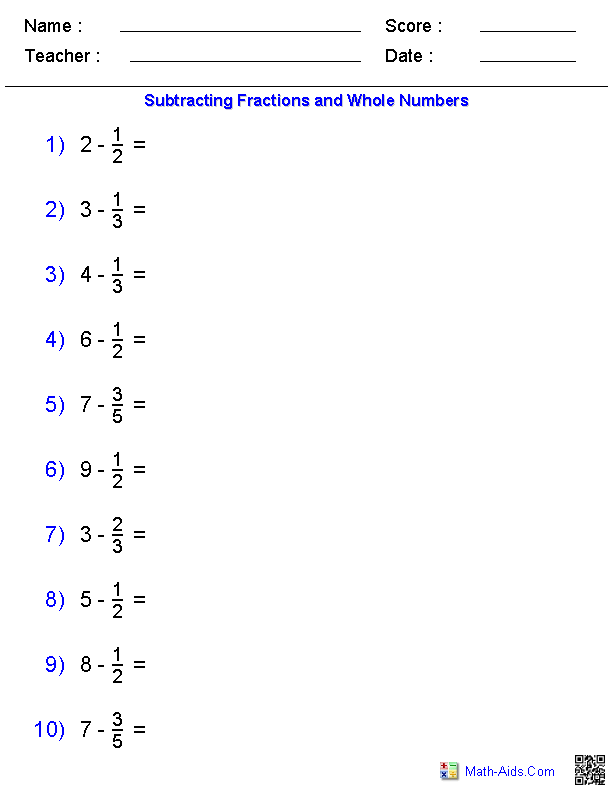##### Subtracting Mixed NumbersFractions Worksheets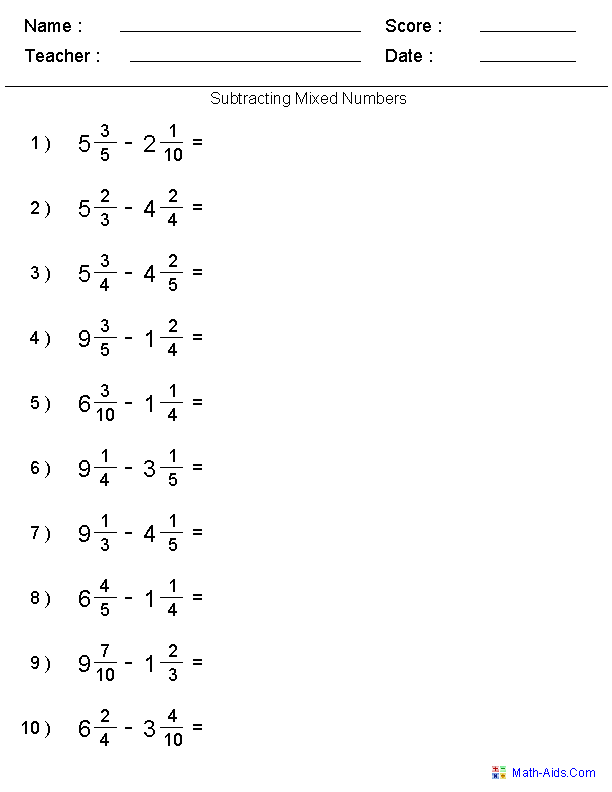##### Adding & SubtractingThree FractionsFractions Worksheets##### Multiplying FractionsFractions Worksheets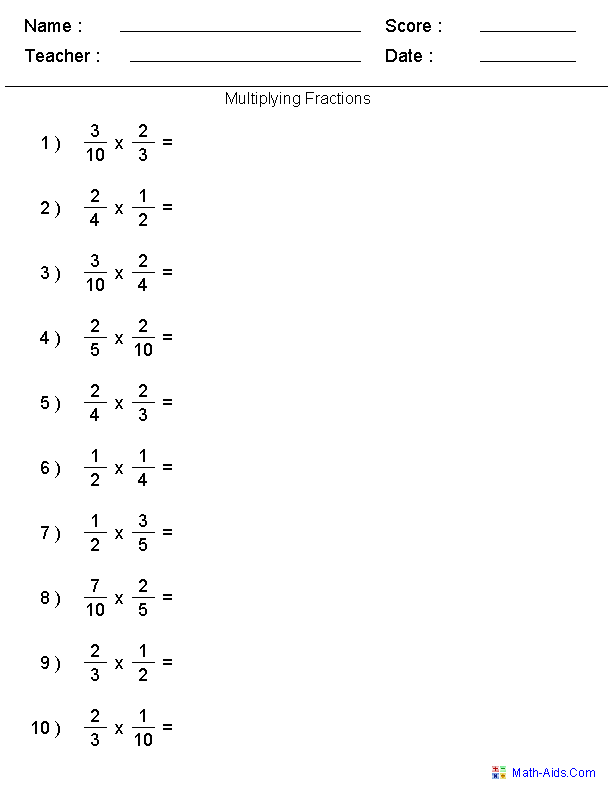##### Multiplying Fractionswith Cross Cancelling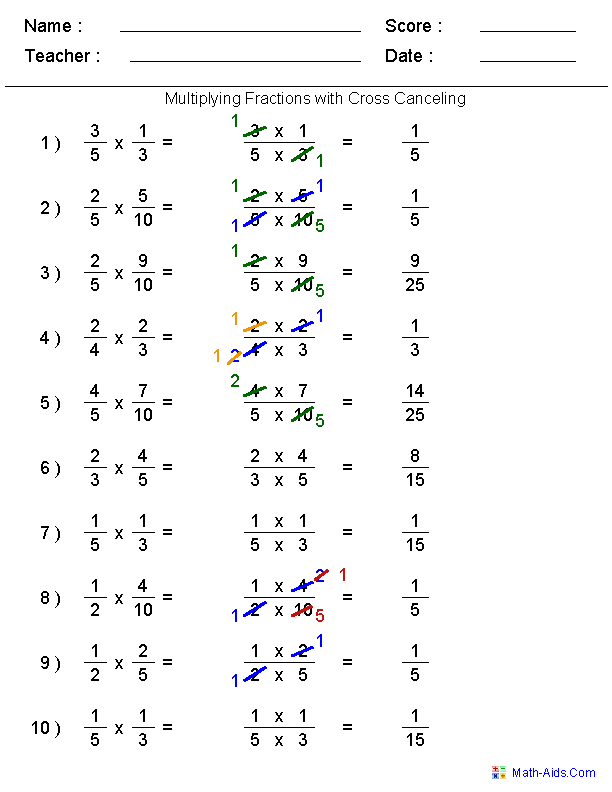##### Multiplying Mixed NumbersFractions Worksheets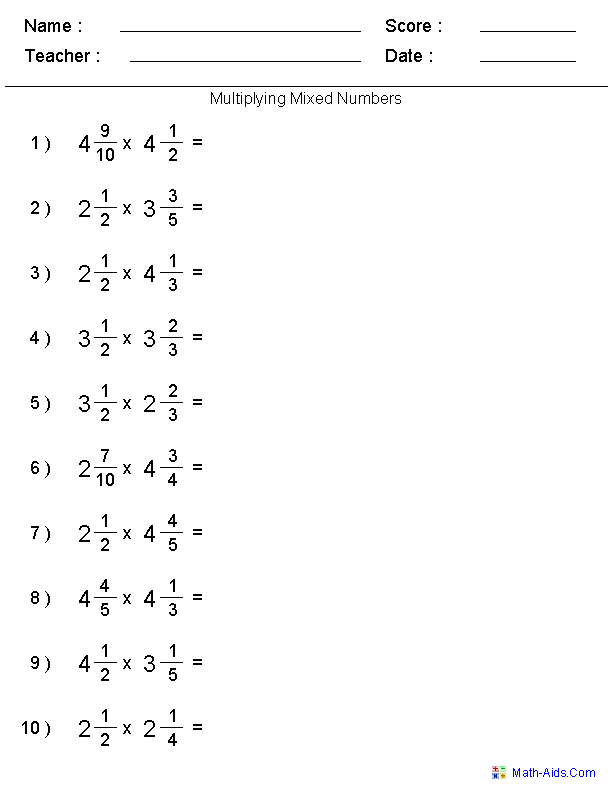##### Multiplying Fractionswith Whole Numbers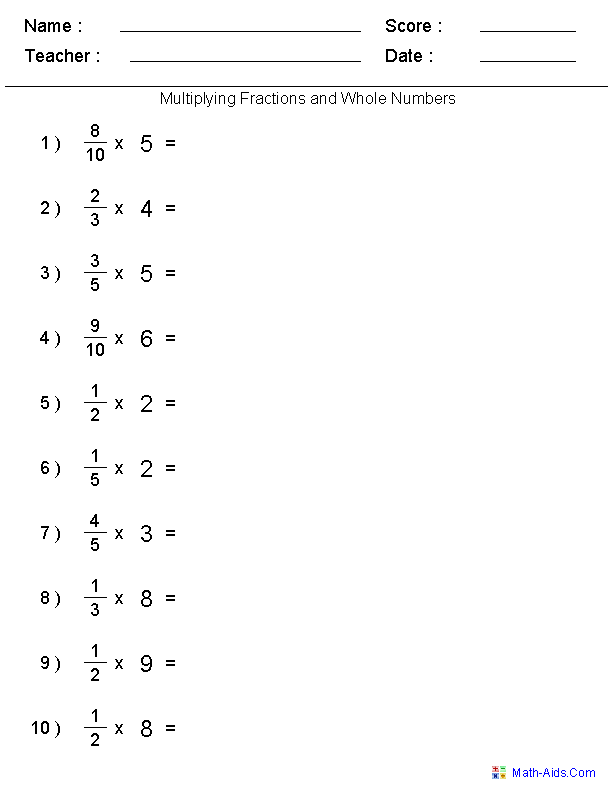##### Dividing FractionsInvert and Multiply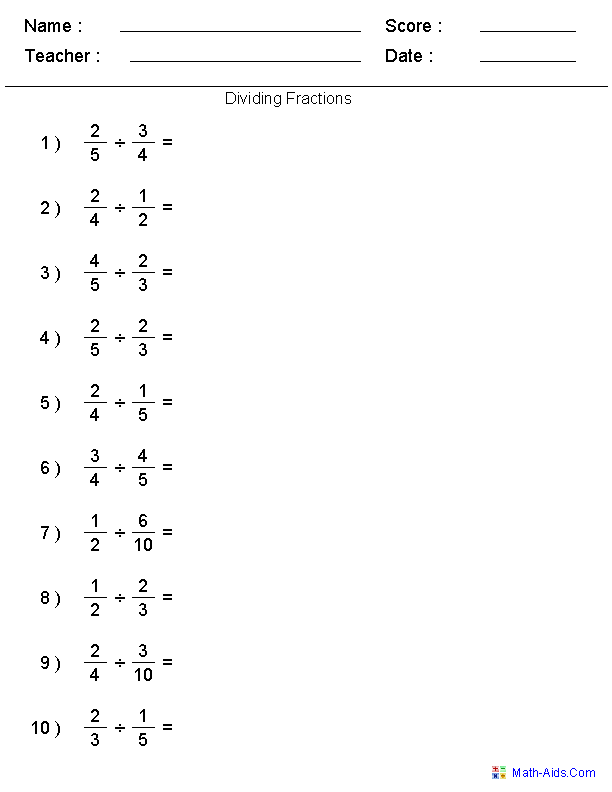##### Dividing Mixed NumbersFractions Worksheets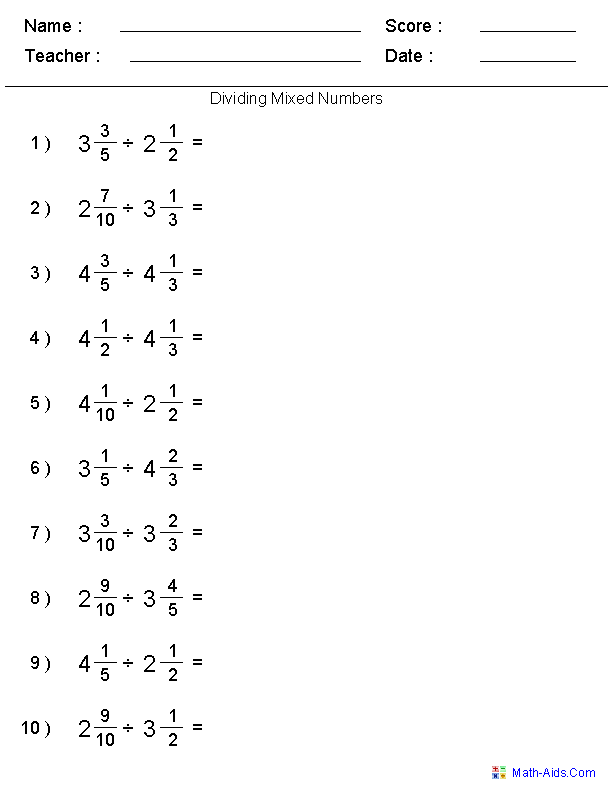##### Dividing Fractionswith Whole Numbers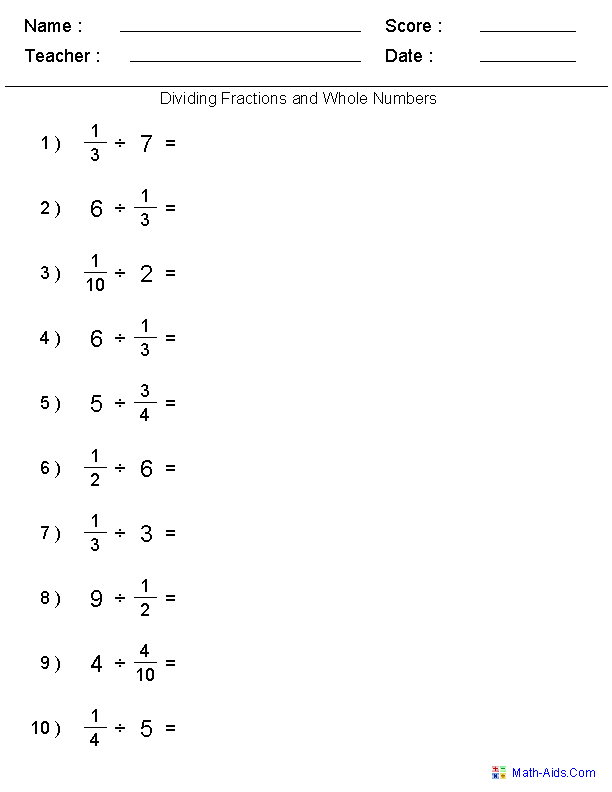##### Prime Factorization TreesFractions Worksheets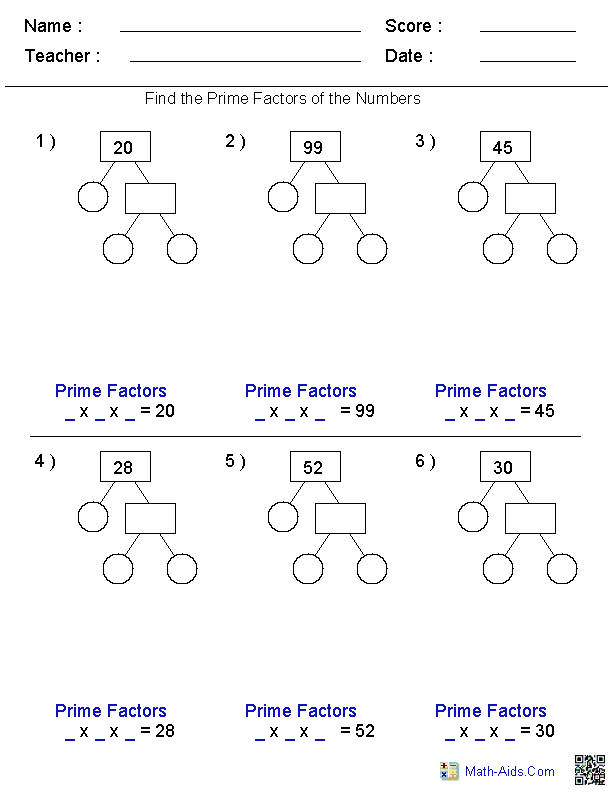##### Greatest Common FactorFractions Worksheets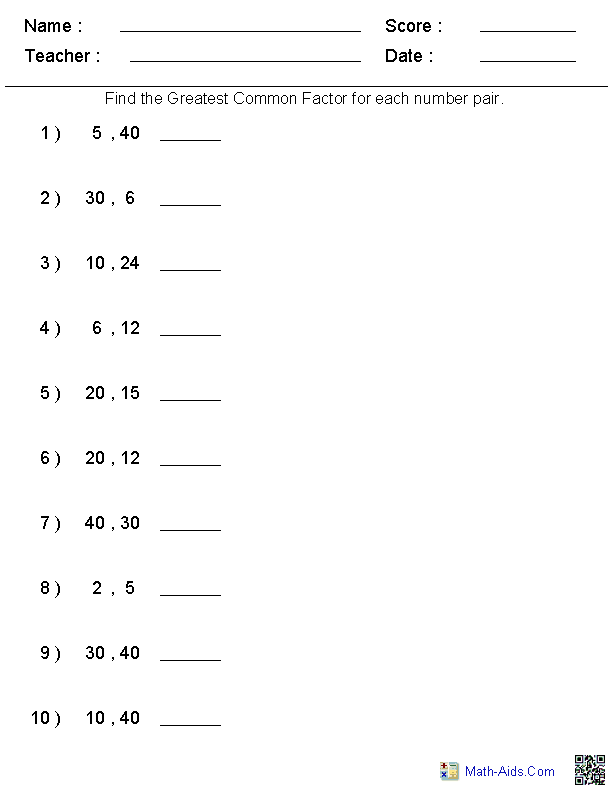##### Least Common MultipleFractions Worksheets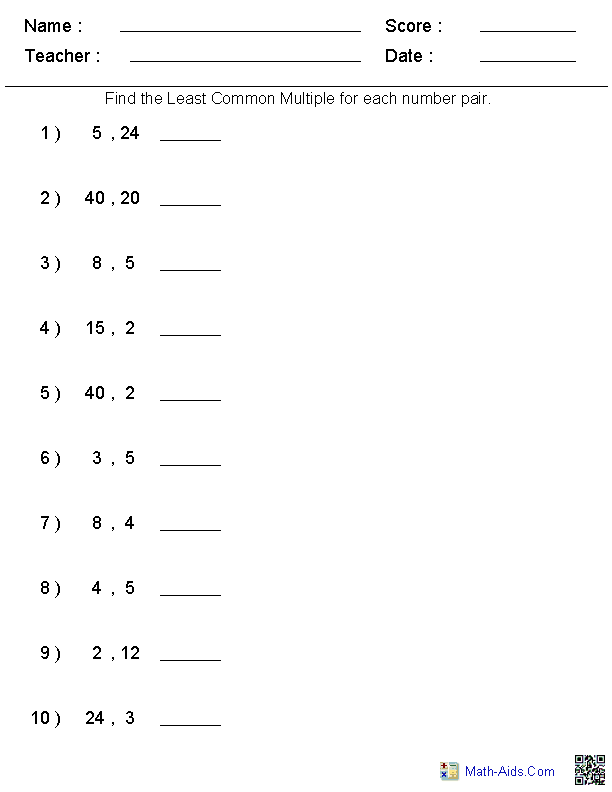##### Equivalent Fraction ProblemsFractions Worksheets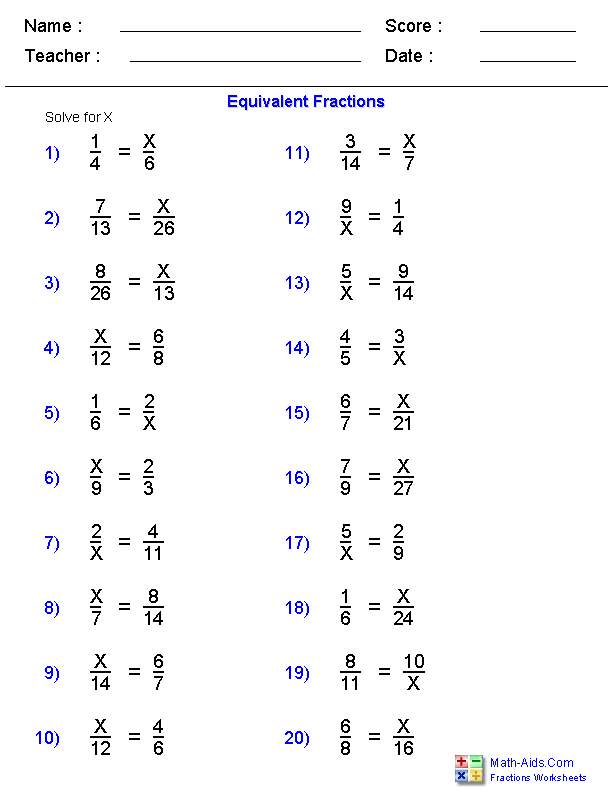##### Equivalent FractionsFractions Worksheets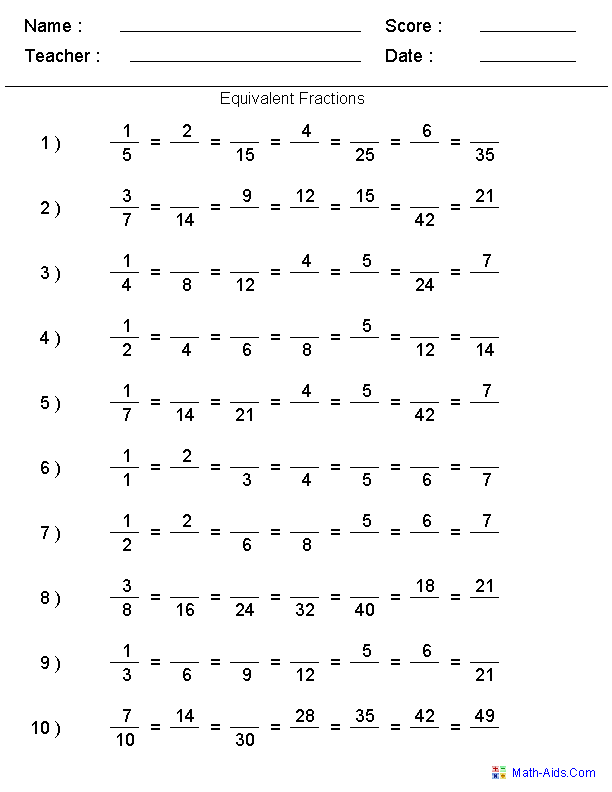##### Reducing FractionsFractions Worksheets##### Converting Between Fractions& Decimals Worksheets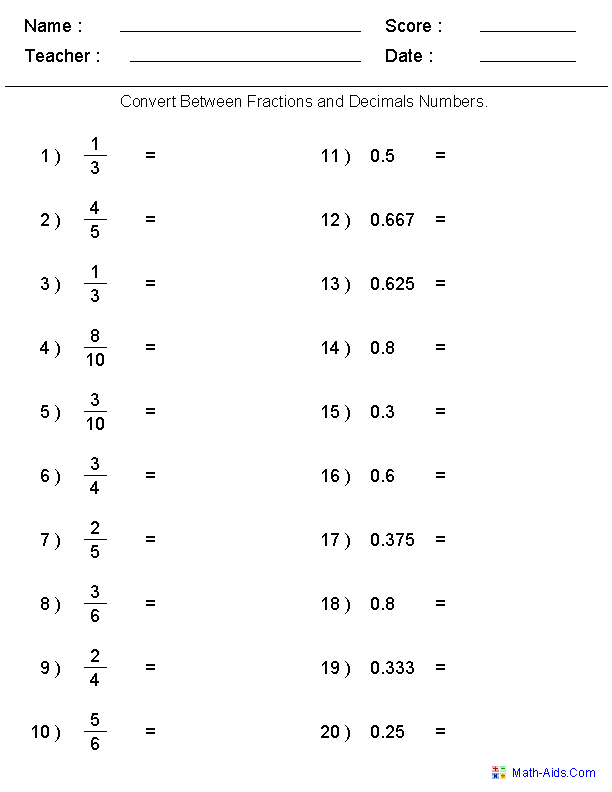##### ComparingFractions Worksheets##### Denominators and NumeratorsComparison Worksheets##### Comparing Fractions& Decimals Worksheets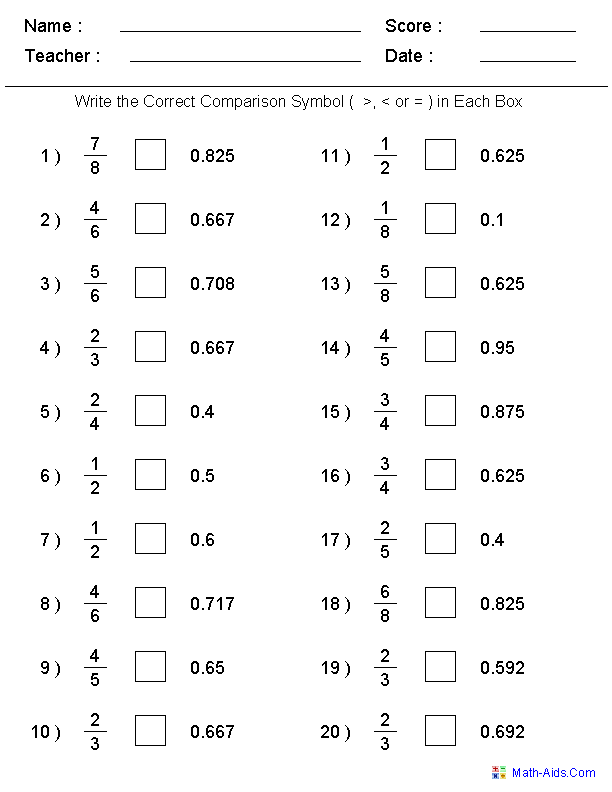##### Improper & Mixed NumberFractions Worksheets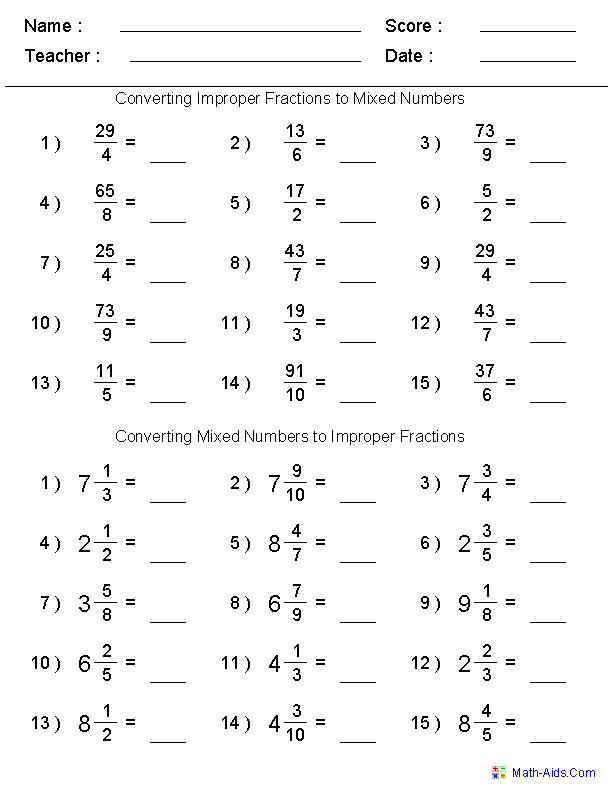##### Solving Fractions withExponents Worksheets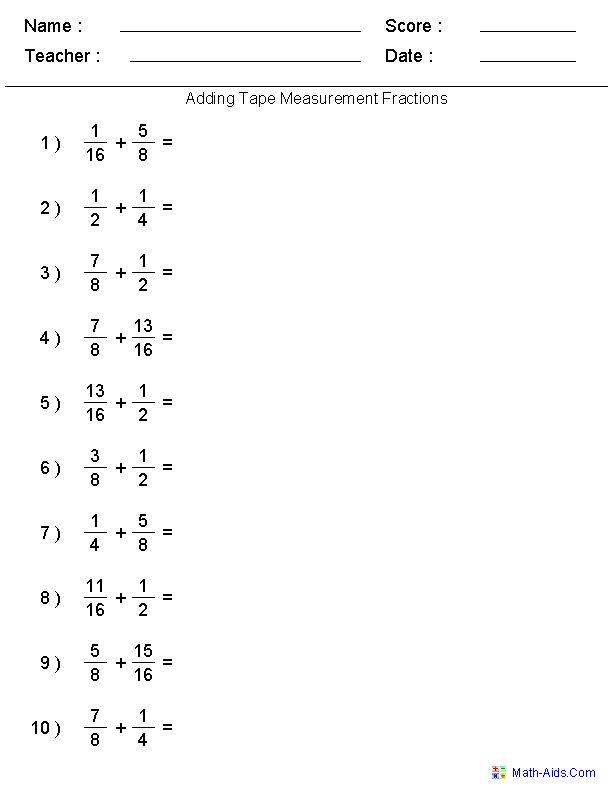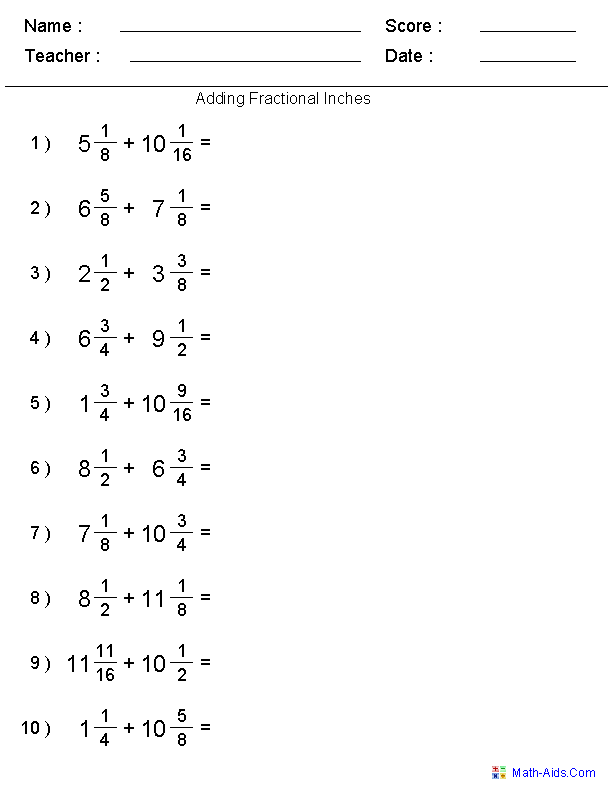##### Subtracting Tape MeasureFractions Worksheets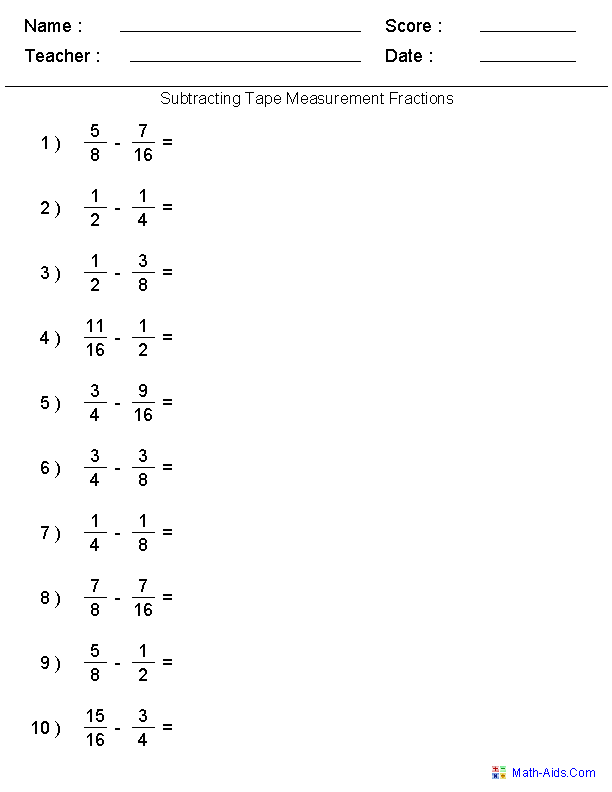##### Subtracting FractionalInches Worksheets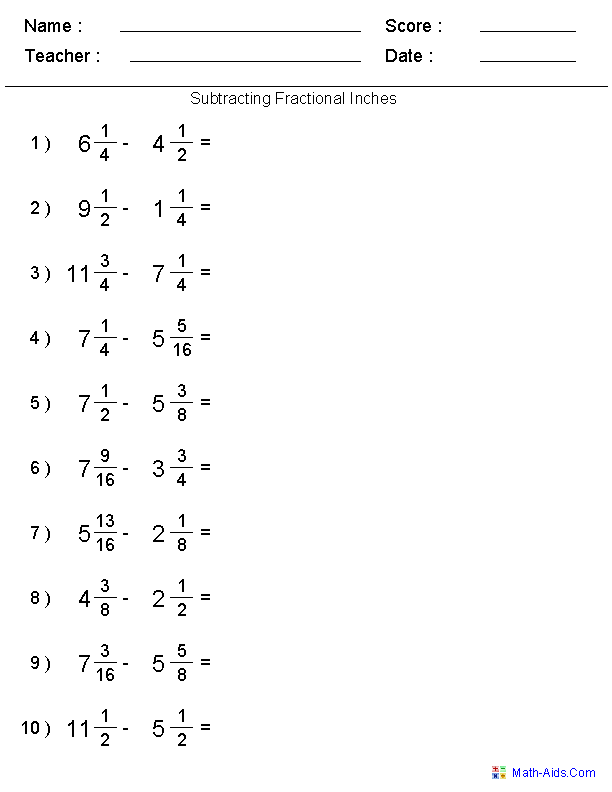##### Decimal Equivalents of Fractionsfor an Inch Worksheets##### Finding Fractionsof Whole NumbersFractions Worksheets##### Adding and SubtractingFractional Incheswith Borrowing Worksheets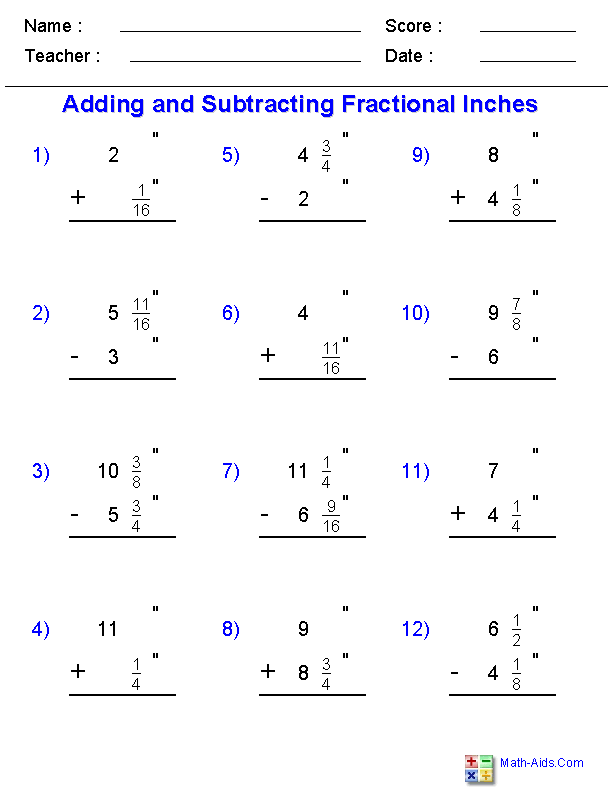##### Adding and SubtractingFractional Feet & Incheswith Borrowing Worksheets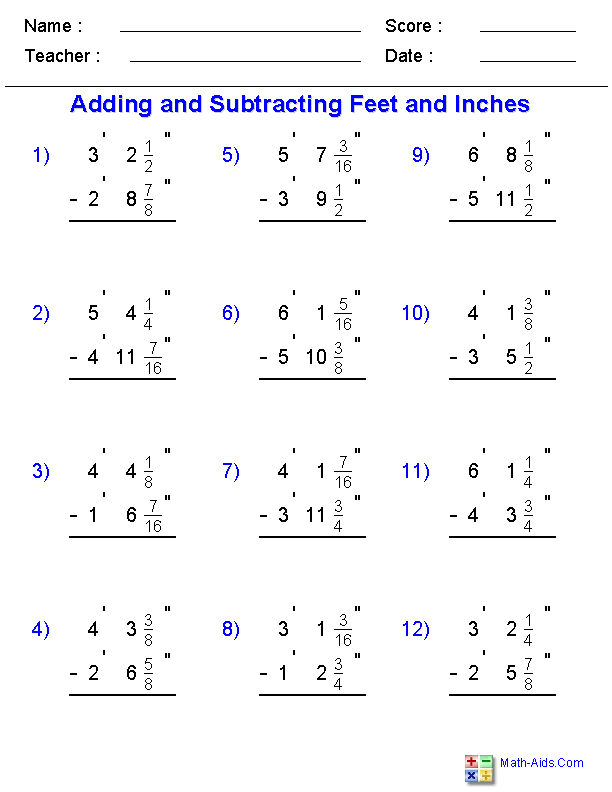##### Ordering FractionsFractions Worksheets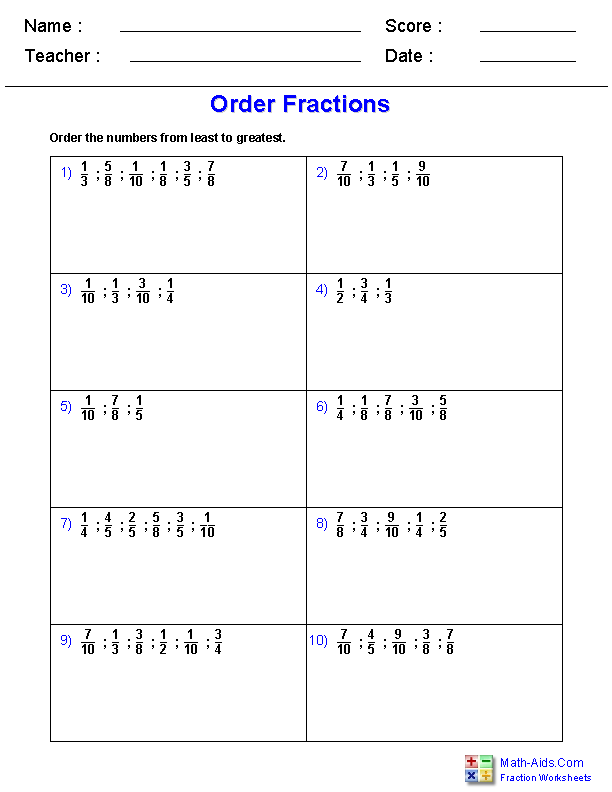Recommended Videos

## Detailed Description for All Fractions Worksheets

Visual Aids for Teaching Fractions Worksheets
Use these fractions worksheets to produce rectangular fractions bars and pie wedge fractions to be used as visuals in your teaching lesson plans. These fractions worksheets will produce fraction representations from a whole thru 1/12's. These fractions worksheets are great teaching aids for teaching your children different fractions. These worksheets are appropriate for Kindergarten, 1st Grade, and 2nd Grade.

Visual Fractions Worksheets
These fractions worksheets are great for teaching different fractions using visual fraction problems. These worksheets will produce fraction representations with denominators of 2 through 12. The students will be asked to identify the fractions for the shaped in shape, and to shade in the shape for the given fraction. These worksheets are appropriate for Kindergarten, 1st Grade, and 2nd Grade.

These fractions worksheets are great practice for beginning to add simple fractions. These fractions problems include visual representations to aid the student in the addition. The fractions will have the same denominators and not exceed the value of one. These worksheets will randomly generate 5 fraction addition problems per worksheet with the answer worksheet.

These fractions worksheets are great practice for beginning to add simple fractions. These fractions problems will have the same denominators and not exceed the value of one. These worksheets will generate 10 fraction addition problems per worksheet.

These fractions worksheets are great for testing children in their adding of two fractions. These fractions worksheets may be selected for five different degrees of difficulty. The answer worksheet will show the progression on how to solve the fraction problems. These worksheets will generate 10 fraction addition problems per worksheet.

These fractions worksheets are great for testing children in their adding of three fractions. These fractions worksheets may be selected for five different degrees of difficulty. The answer worksheet will show the progression on how to solve the fraction problems. These worksheets will generate 10 fraction addition problems per worksheet.

These fractions worksheets are great for practicing Adding Mixed Number Fractions Problems. The fractions worksheets may be selected for five different degrees of difficulty. The answer worksheet will show the progression on how to solve the problems. These worksheets will generate 10 fraction mixed number addition problems per worksheet.

Visually Subtracting Simple Fractions Worksheets
These fractions worksheets are great practice for beginning to subtract simple fractions. These fractions problems will have the same denominators and not equal zero. These worksheets will randomly generate 5 fraction subtraction problems per worksheet with the answer worksheet.

Subtracting Simple Fractions Worksheets
These fractions worksheets are great practice for beginning to subtract simple fractions. These fractions problems will have the same denominators and not equal zero. These worksheets will randomly generate 10 fraction subtraction problems per worksheet with the answer worksheet.

Subtracting Fractions Worksheets
These fractions worksheets will produce problems that will test children in their subtraction of two fractions. The fractions worksheets may be selected for five different degrees of difficulty. The answer worksheet will show the progression on how to solve the problems. These worksheets will randomly generate 10 fraction subtraction problems per worksheet with the answer worksheet.

Subtracting Three Fractions Worksheets
These fractions worksheets are great for testing children in their subtracting of three fractions. These fractions worksheets may be selected for five different degrees of difficulty. The answer worksheet will show the progression on how to solve the fraction problems. These worksheets will generate 10 to 100 fraction subtraction problems per worksheet.

Subtracting Fractions and Whole Numbers Worksheets
These fractions worksheets are perfect for practicing subtracting fractions from whole numbers. You can select from five different degrees of difficulty. The easiest will keep the denominators and the numerators between 1 and 9. The hardest will keep the numerators between 1 and 20. The answer worksheet will show the progression on how to solve the problems. These fraction worksheets will generate 10 or 15 problems per worksheet.

Subtracting Mixed Number Fractions Worksheets
These fractions worksheets are great for practicing subtracting Mixed Number Fraction Problems. You may select whether or not the fractions worksheets require regrouping or not. The fractions worksheets may be selected for five different degrees of difficulty. The answer worksheets will show the progression on how to solve the problems. These worksheets will generate 10 or 15 mixed number subtraction problems per worksheet.

Adding & Subtracting Three Fractions Worksheets
These fractions worksheets are great for testing children in their adding and subtracting of three fractions. These fractions worksheets may be selected for five different degrees of difficulty. The answer worksheet will show the progression on how to solve the fraction problems. These worksheets will generate 10 to 100 fraction problems per worksheet.

Multiplying Fractions Worksheets
These fractions worksheets are great for working on multiplying fractions. The fractions worksheets may be selected for three different degrees of difficulty. The answer worksheets will show the progression on how to solve the problems. These worksheets will generate 10 fraction multiplication problems per worksheet.

Multiplying Fractions Worksheets with Cross Cancelling
These fractions worksheets are great for working on multiplying fractions. The fractions worksheets may be selected for four different degrees of difficulty. The answer worksheets will show the progression on how to solve the problems. These worksheets will generate 10 fraction multiplication problems per worksheet.

Multiplying Mixed Number Fractions Worksheets
These fractions worksheets are great for practicing Multiplying Mixed Numbers Problems. The fractions worksheets may be selected for two different degrees of difficulty. The answer worksheets will show the progression on how to solve the problems. These worksheets will generate 10 fraction mixed number multiplication problems per worksheet.

Multiplying Fractions Worksheets with Whole Numbers
These fractions worksheets are great for working with multiplying fractions with Whole Numbers. The fractions worksheets may be selected for three different degrees of difficulty. The answer worksheets will show the progression on how to solve the problems. These worksheets will generate 10 fraction multiplication problems per worksheet.

Dividing Fractions Worksheets | Invert and Multiply
These fractions worksheets are great for working on dividing fractions. The fractions worksheets may be selected for three different degrees of difficulty. The answer worksheets will show the progression on how to solve the problems. These worksheets will generate 10 fraction division problems per worksheet.

Dividing Mixed Number Fractions Worksheets
These fractions worksheets are great for practicing Dividing Mixed Numbers Problems. The fractions worksheets may be selected for two different degrees of difficulty. The answer worksheets will show the progression on how to solve the problems. These worksheets will generate 10 fraction mixed number division problems per worksheet.

Dividing Fractions and Whole Numbers Worksheets
These fractions worksheets are great for working with dividing fractions and Whole Numbers. The fractions worksheets may be selected for two different degrees of difficulty. The answer worksheets will show the progression on how to solve the problems. These worksheets will generate 10 fraction division problems per worksheet.

Prime Factorization Trees Worksheets
These fractions worksheets are great for practicing finding all of the prime factors contained in a number. The Prime Factorization Trees Worksheets are great visual aids. These fractions worksheets may be selected from two different degrees of difficulty. These worksheets will generate 6 Prime Factorization Tree problems per worksheet and the answer keys are generated.

Greatest Common Factor Worksheets
These fractions worksheets are great for practicing finding the Greatest Common Factor of number sets. Understanding Greatest Common Factors are very important for working with fraction problems. These fractions worksheets may be selected from four different number ranges. These worksheets will generate 10, 15 or 20 Greatest Common Factor problems per worksheet.

Least Common Multiple Worksheets
These fractions worksheets are great for practicing finding the Least Common Multiple of number sets. Understanding Least Common Multiples are very important for working with fraction problems. These fractions worksheets may be selected from four different number ranges. These worksheets will generate 10, 15 or 20 Least Common Multiple problems per worksheet.

Equivalent Fraction Problems Worksheets
These fractions worksheets will produce equivalent fraction problems with different numerators and denominators. You may select between 10, 15, 20 or 30 problems for each worksheet.

Equivalent Fractions Worksheets
These fractions worksheets have rows of equivalent fractions, each with either the numerator or denominator left blank. One fraction in each row will be written with both the numerator and denominator. The student will fill in the missing numerators and denominators. These worksheets will generate 10 Equivalent Fractions problems per worksheet.

Reducing Fractions Worksheets
These fractions worksheets are great for testing children in their reducing of fractions. These fractions worksheets may be selected from easy, medium or hard level of difficulty. You may select 10, 20 or 30 problems per worksheets.

Converting Between Fractions and Decimals Worksheets
These fractions worksheets are great for testing children for converting between Fractions and Decimals. You may select 3 or 4 digit to the right of the decimal. These worksheets will create twenty problems per page.

Comparing Fractions Worksheets
These fractions worksheets are great for testing children to compare Fractions to see if they are greater than, less than or equal. These worksheets may be selected for different denominators so the problems may be positive, negative or mixed.

Denominators and Numerators Comparison Worksheets
These fractions worksheets are great for testing children in their comparison of fractions with similar denominators and numerators to see if they are greater than or less than. You may select different denominators and have the problems produce similar denominators, or problems with similar numerators, or a mixture of both.

Comparing Fractions and Decimals Worksheets
These fractions worksheets are great for testing children to compare Fractions and Decimals to see if they are greater than, less than or equal. These worksheets may be selected for different denominators so the problems may be positive, negative or mixed.

Converting Improper Fractions and Mixed Numbers Worksheets
These fractions worksheets are great for working on converting Improper Fractions and Mixed Numbers. These fractions worksheets may be selected from easy, medium or hard level of difficulty. It will produce 15 Improper Fractions problems and 15 Mixed Number problems per worksheets.

Solving Fractions with Exponents Worksheets
These fractions worksheets are great for practicing solving fractions with exponents. These worksheets will generate 20 fractions problems with exponents per worksheet.

These fractions worksheets are great for practicing how to add measurement you would find on a tape measure. These fractions worksheets will use 1/2's, 1/4's, 1/8's. 1/16's and there is an option to select 1/32's and 1/64's. These worksheets will generate 10 tape measurement fraction addition problems per worksheet.

These fractions worksheets are great for practicing how to add fractional inch measurements that you would find on a tape measure. These fractions worksheets will use 1/2's, 1/4's, 1/8's. 1/16's and there is an option to select 1/32's and 1/64's. These worksheets will generate 10 fractional inch problems per worksheet.

Subtracting Tape Measure Fractions Worksheets
These fractions worksheets are great for practicing how to subtract measurement you would find on a tape measure. The problems will use 1/2's, 1/4's, 1/8's. 1/16's and there is an option to select 1/32's and 1/64's. These worksheets will generate 10 tape measurement fraction subtraction problems per worksheet.

Subtracting Fractional Inches Worksheets
These fractions worksheets are great for practicing how to subtract fractional inch measurements that you would find on a tape measure. These fractions worksheets will use 1/2's, 1/4's, 1/8's. 1/16's and there is an option to select 1/32's and 1/64's. These worksheets will generate 10 fractional inch problems per worksheet.

Decimal Equivalents of Fractions for an Inch Worksheets
These fractions worksheets are great handouts for student learning about fractions and the decimal equivalents for an inch. They will produce a table of all the fractions from 1/64th to 63/64th of an inch.

Finding Fractions of Whole Numbers Worksheets
These Fractions Worksheets are great for testing children for finding fractions of whole numbers. You may select the denominators of the fractions and the type of the problems. The worksheet will create twenty problems per page.

Adding and Subtracting Fractional Inches with Borrowing Worksheets
These Fractions Worksheets are great for practicing how to add, subtract and borrow with fractional inch measurements that you would find on a tape measure. You may select the types of expressions used, the type of operations and the denominators used in the fractions.

Adding and Subtracting Fractional Feet & Inches with Borrowing Worksheets
These Fractions Worksheets great for practicing how to add, subtract and borrow feet and fractional inch measurements that you would find on a tape measure. You may select the types of expressions used, the type of operations and the denominators used in the fractions.

Ordering Fractions Worksheets
These Fractions Worksheets will produce problems that involve ordering fractions. The student will be given a list of fractions and be asked to order them in ascending or descending order. You may select the number of problems per worksheets, the number of fractions to sort per problem, the range of numerators and denominators, as well as the way to order the fractions.